Published

# Bicycle Movement Tracking Using Particle Photon

Track the distance, speed, and acceleration of your bicycle in real time using two Particle Photons.

IntermediateFull instructions provided10 hours1,301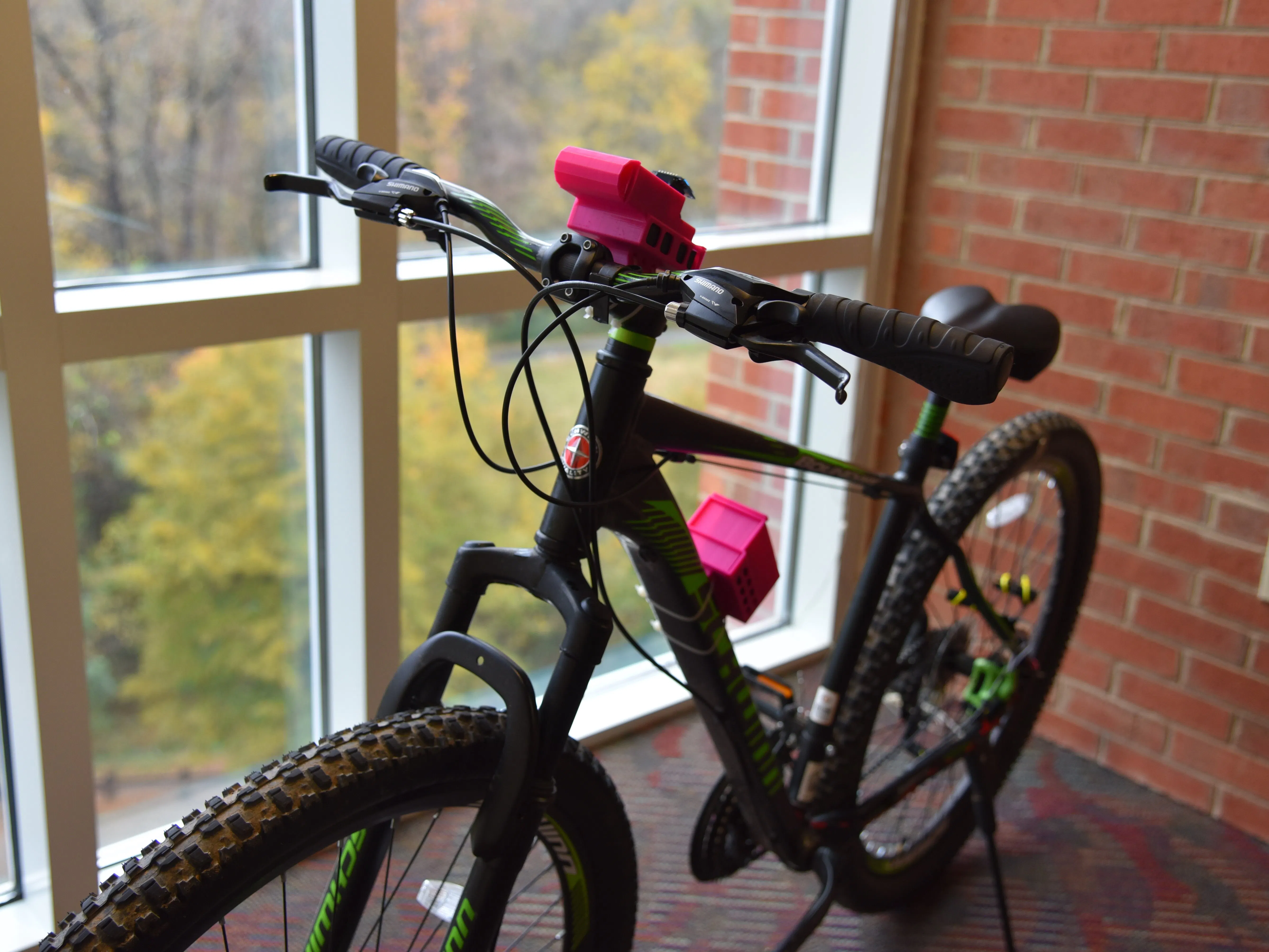## Things used in this project

### Hardware componentsParticle Photon
×2
 7Pin 0.96 Inch IIC/SPI Serial 128x64 White OLED Display Module
×1×2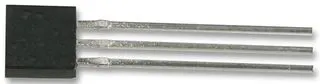Hall Effect Sensor
×1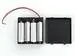4xAA battery holder
×2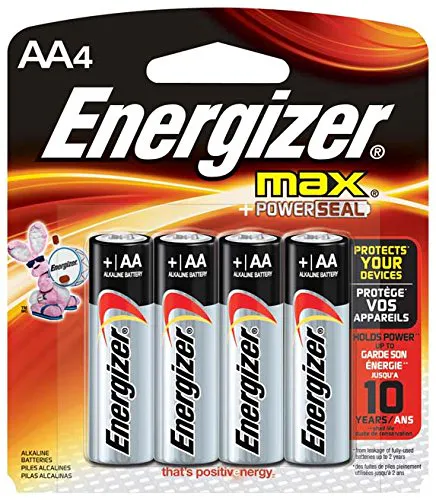AA Batteries
×2
 Electrical Tape
×1
 Magnets
×2Resistor 1k ohm
×3Resistor 10k ohm
×1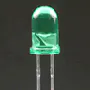5 mm LED: Green
×1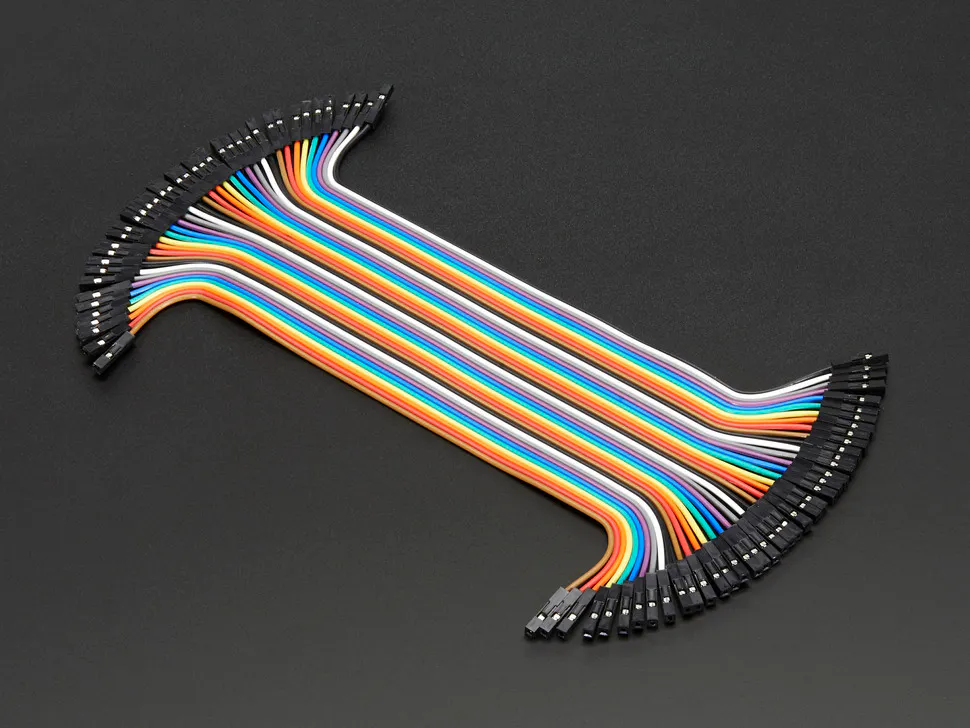Female/Female Jumper Wires
×1
 Male/Male Jumper Wires
×1

### Software apps and online servicesThingSpeak API

### Hand tools and fabrication machines

 Wire Strippers
 Weller WLC100 40-Watt Soldering Station
 3D Printer
 Superglue
 Zip Ties

## Custom parts and enclosures

### Speedometer Housing

Autodesk Inventor File for the Speedometer Mount. The mount contains a particle photon, battery pack, and OLED display. The neck is adjusted on a per bicycle basis, so the radius may need to be changed for a different bicycle.The model has been parametrically modeled in such a way so that editing the radius drives relations for other parts of the mount while allowing the housing the remain the same size. Depending on the display used, a spacing block may need to be superglued to the mount to allow for adequate wiring. It is advised to bundle extra wires together with zip-ties for space management inside of the housing. Lastly, the mount is secured with zip-ties.

### Data Acquisition Photon Housing

Contains the housing for the Particle Photon and Battery pack used for the Hall Effect Sensor. The mount is to be used on a flat surface on the bike and zip-tied down. There are three holes in the housing for wire management. The additional housing space is intended for the addition of other sensors if desired. These can be used for the hall effect sensor, indicator LED, and battery power supply

## Schematics

### Hall Effect Sensor Module [Breadboard View]

Breadboard view of the Hall Effect Sensor Module Photon Setup.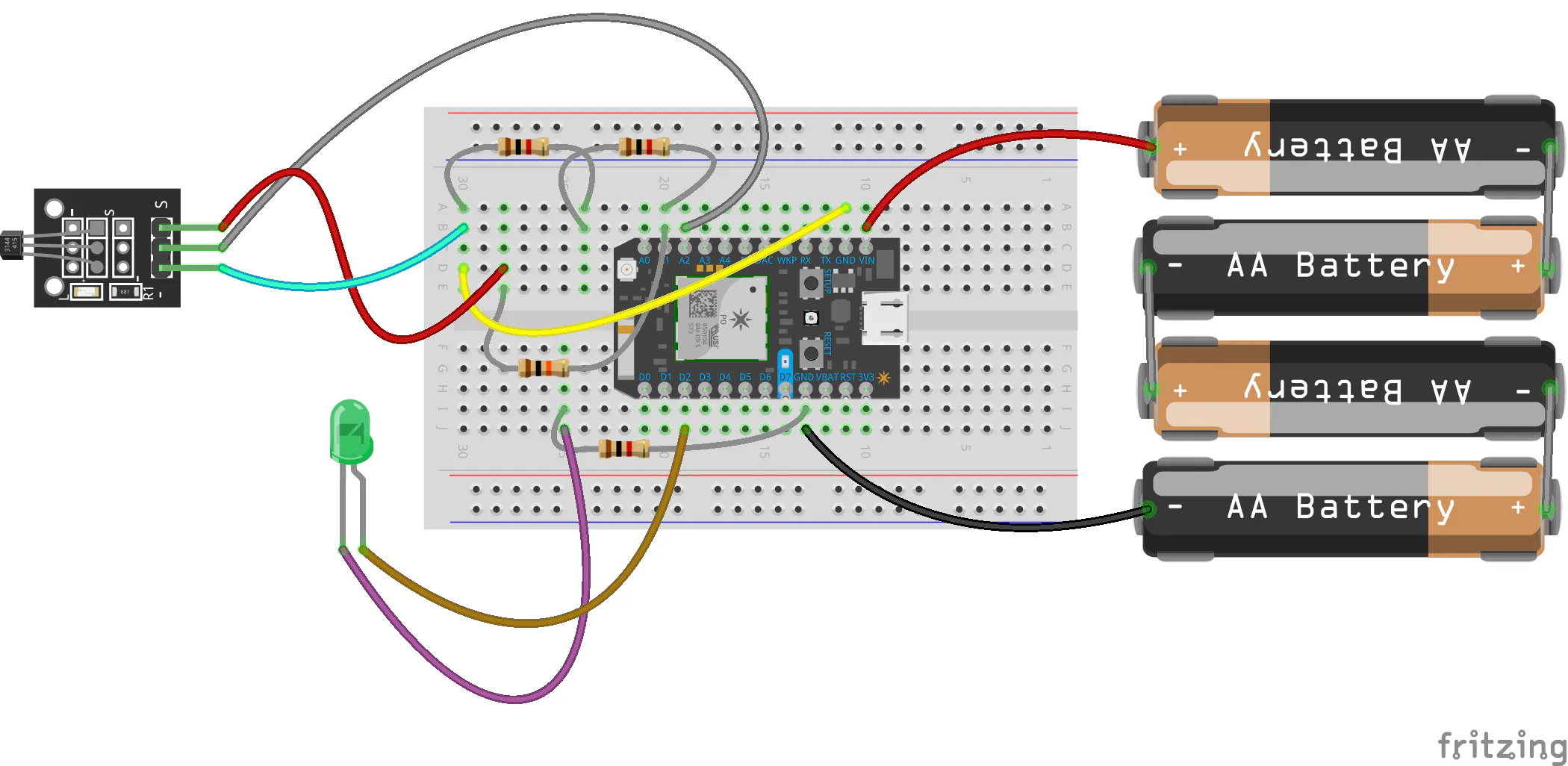Breadboard view of the Display Module Photon Setup.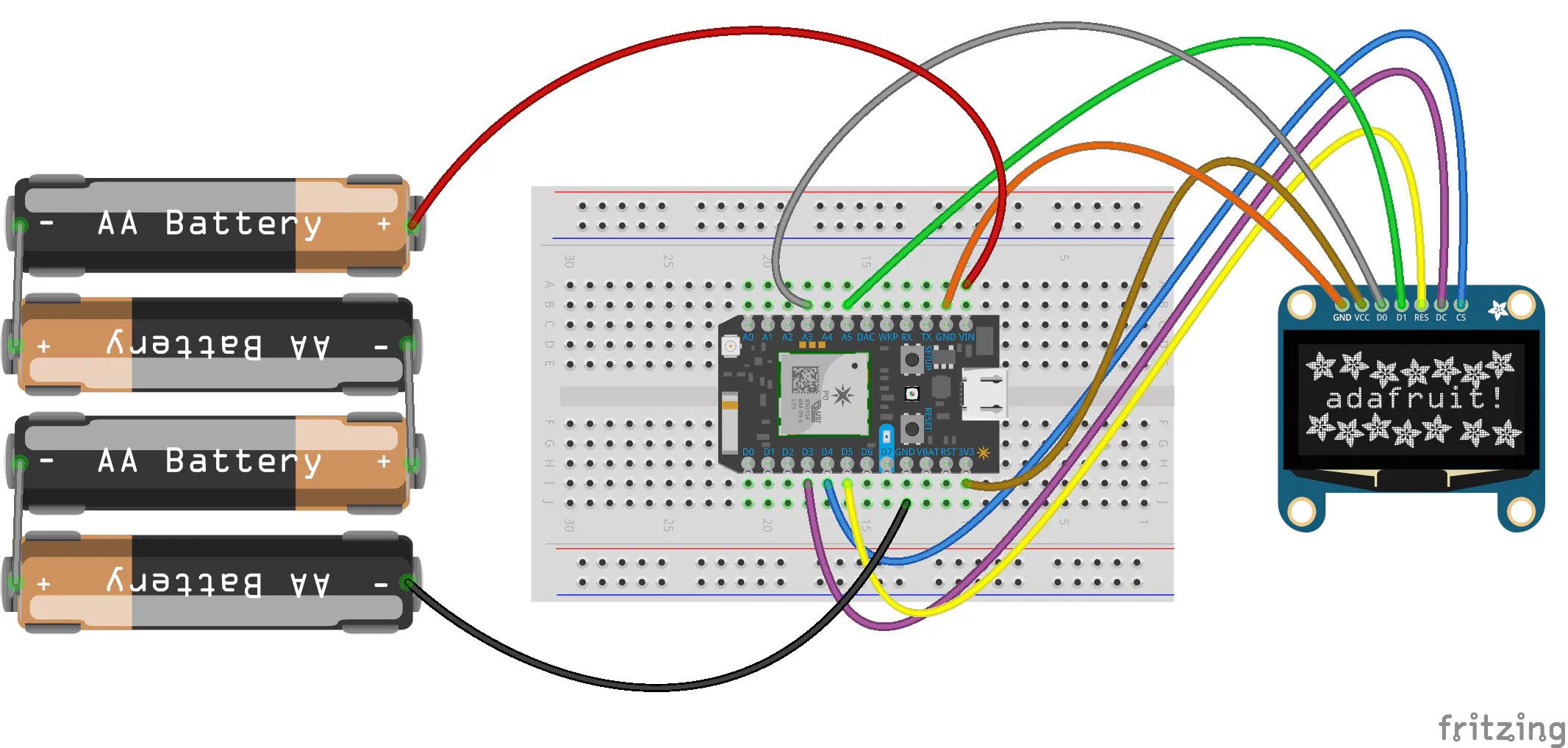### Hall Effect Sensor Module [Circuit Diagram]

Circuit Diagram of the Hall Effect Sensor Module Photon Setup.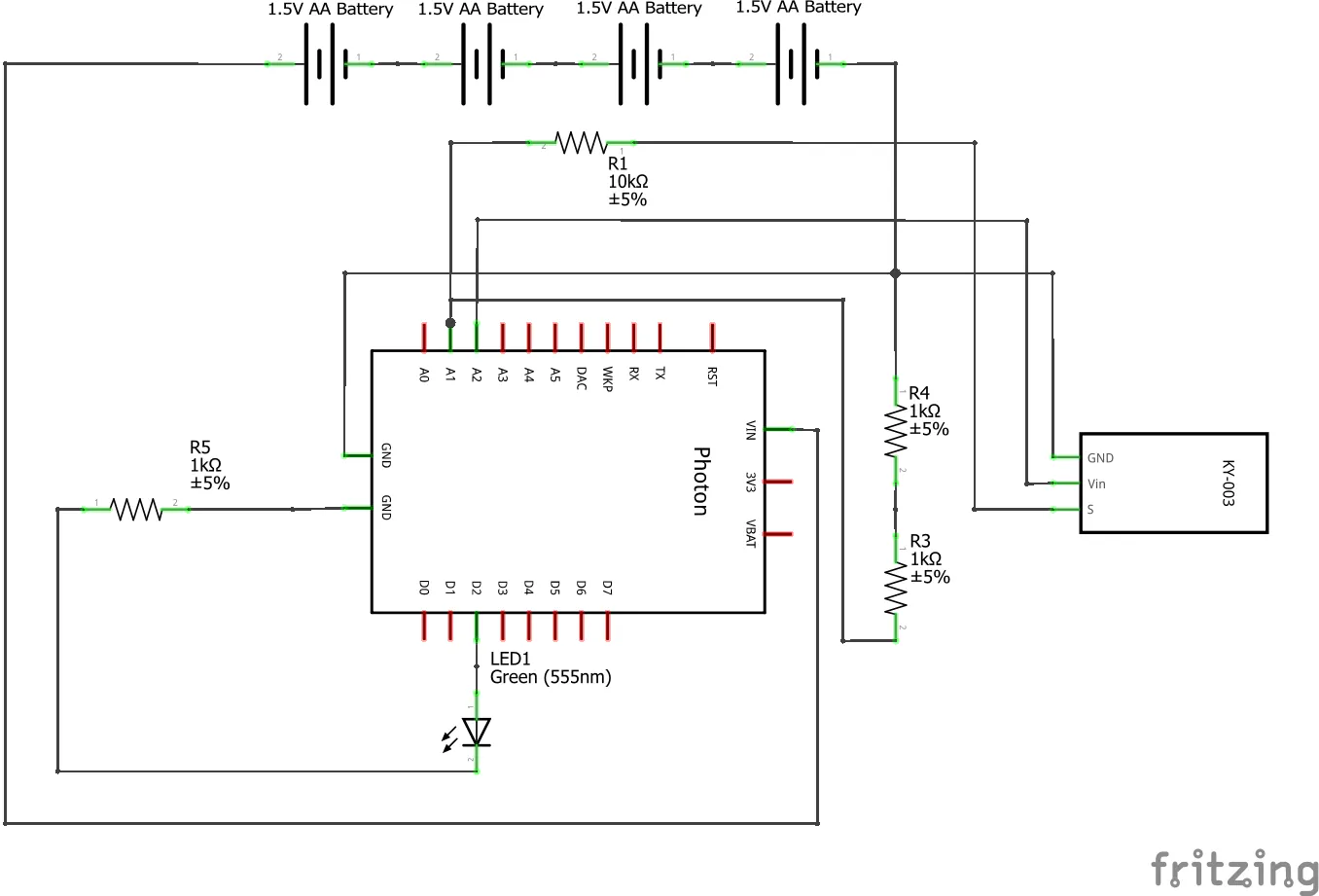### Display Module [Circuit Diagram]

Circuit Diagram of the Display Module Photon Setup.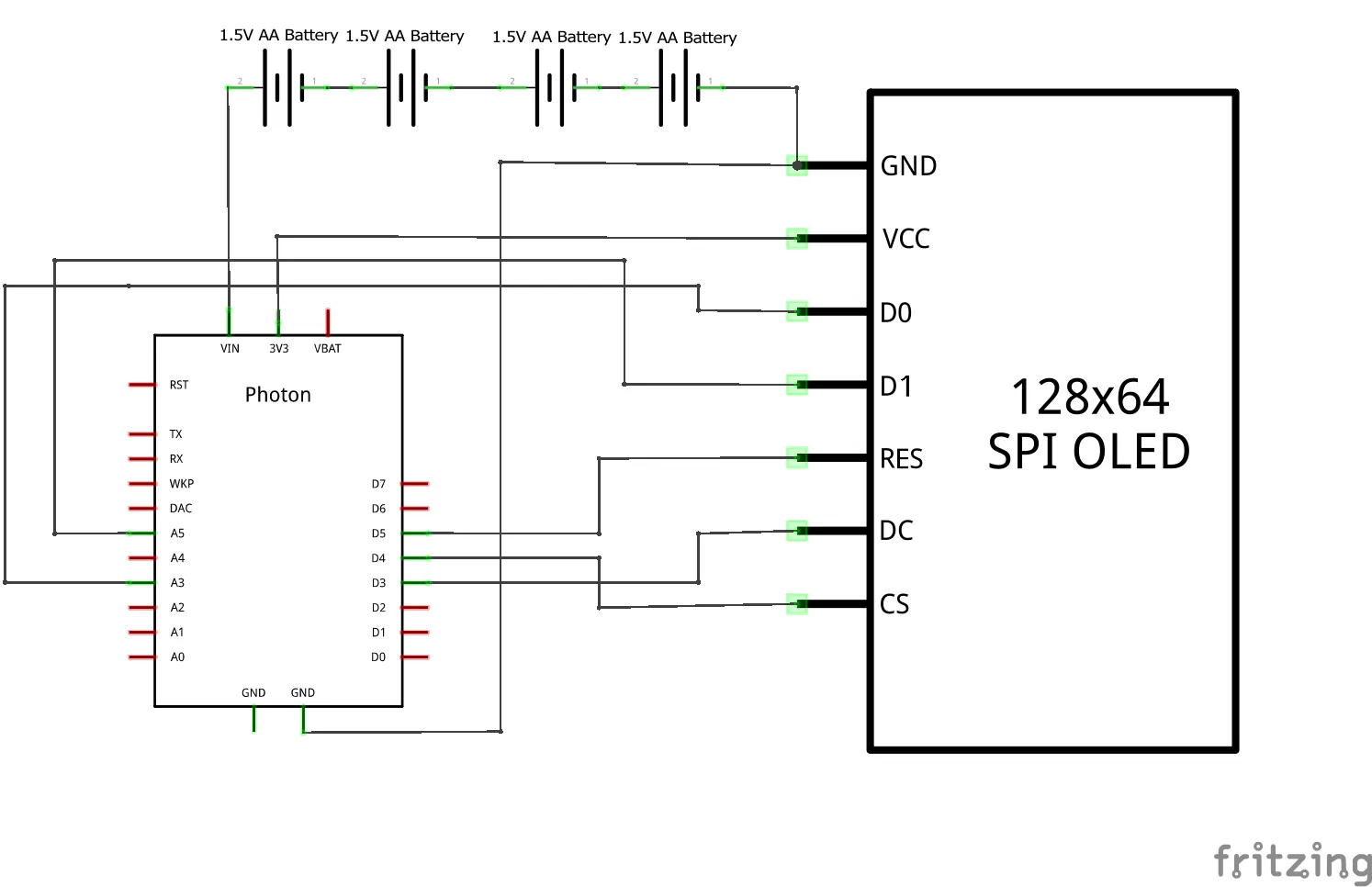## Code

### Hall Effect Sensor Module Photon Code

C/C++
This is the code used in the Hall Effect Module Photon for determining the speed of the bike based on the data from the hall effect sensor. From this data, the number of passes is determined and is adjusted for the distance traveled per rotation of the bike tires. The velocity data is sent to the Display Module and Thingspeak via Particle.Publish. This code also checks to see if the Display Module is turned on via
Particle.Subscribe and turns on the Led upon detection of the Display Module being online and turns off upon no indicators for 11 seconds.
```// -------------------------------------------------------
// Hall Effect Sensor Module Proceedure
// By: William Ladd and Dan Cornett
//
// MEGR3171 Fall 2018 IOT Project
//
//
//
// -------------------------------------------------------

// Declare all Pins
int power = A2;
int sig = A1;
int ledpower = D2;

// Declare all Integers, Floats, and Character Arrays
int looptime;
int calculation;
int magnettime;
int passsingle;
int passamt;
int passloga;
int passlogb;
int passlogc;
int totaltostart;
int cyc;
int subcyc;
int timesumA;
int timesumB;
int timesumC;
int timecheckA;
int timecheckB;
int timecheckC;
int timepass;
int lightcheck;
int cycpassamt;
float calibration;
int magcount;
int timesincemag;
float simplefloat;
float calculatedvelocity;
char cldsubmit;

void setup() {

// Setup Pin Modes
pinMode(power,OUTPUT);
pinMode(ledpower,OUTPUT);
pinMode(sig,INPUT);

// Turn on power to Hall Effect Sensor
digitalWrite(power,HIGH);
// Turn off power for Led Connection Indicator
digitalWrite(ledpower,LOW);

// Declare variables for monitoring from particle console
Particle.variable("calculation", &calculation, INT);
Particle.variable("singlepass", &passsingle, INT);
Particle.variable("timeinloop", &looptime, INT);
Particle.variable("passamount", &passamt, INT);
Particle.variable("magnettime", &magnettime, INT);
Particle.variable("totaltostart", &totaltostart, INT);

// Declare function for ability to turn off and on power to Hall Effect Sensor
Particle.function("power",powerToggle);

// Setup Hook Response for Thingspeak
// Subscribe to Status indicator for Display Module

// Setup Default Variable Values
calculation = 0;
magnettime = 0;
passamt = 0;
subcyc = 0;
timesincemag = 0;

// Enter the distance traveled per rotation of the wheel in inches into the
// calibration value to calculate bike velocity.
calibration = 91.000;
// Enter the number of magnets used on the wheel
magcount = 4;
}

void loop() {

// Gather output data from Hall Effect Sensor
looptime = (looptime + 1);
// Add 1 to loop time tracking counter

timesincemag = timesincemag + 2;

if (looptime>=550) {
// Check if loop time is 550 ((2ms delay per loop -> 1.100s between critical times due to publish limits of photon)

subcyc = subcyc + 1;

if (subcyc==4) {subcyc = 1;} else {}

if (subcyc==1) {timepass = timesumA; timesumA = 0; timecheckA = 0;} else {}
if (subcyc==2) {timepass = timesumB; timesumB = 0; timecheckB = 0;} else {}
if (subcyc==3) {timepass = timesumC; timesumC = 0; timecheckC = 0;} else {}
if (timepass==0) {timepass=100000000;} else {}

cyc = (cyc + 1);
// Increment the cycle number
cycpassamt = cycpassamt + passamt;
// Add together number of passes during this set of 15 cycles
if (lightcheck<=0) {
// Check for Disconnect of Display Photon, Turn off Led if Disconnected else Increment down Disconnect Timer
digitalWrite(ledpower,LOW);
}
else {
lightcheck = (lightcheck - 1);
}

if (cyc>=15) {
// Check which number cycle this is, every 15 cycles, on cycle number 15,
// publish to Thingspeak, else publish to Display

looptime = 0;
passlogc = passlogb;
passlogb = passloga;
passloga = passamt;
// Memorize Passes Amount of Last 2 Periods of 1.1 Seconds Each
// Overwrite each previous one with each newer one
simplefloat = cycpassamt;
// Retrieve Data of last 16.5 seconds and convert to Float type for calculations
calculatedvelocity = (simplefloat*(calibration/(magcount*16.5*5280*12/3600)));
// Calculate the average velocity in MPH over the past 16.5 seconds with
// 2 magnets per rotation for submission to Thingspeak

Serial.begin();
// Submit data to Thingspeak

// Reset cycle number and tracking variables
cycpassamt = 0;
cyc = 0;
if (calculation>=1) {
passsingle = 1;
}
else {
passsingle = 0;
}
calculation = 0;
magnettime = 0;
passamt = 0;

}
else {

looptime = 0;
passlogc = passlogb;
// Memorize Passes Amount of Last 2 Periods of 1.1 Seconds Each
passlogb = passloga;
// Overwrite each previous one with each newer one
passloga = passamt;
passamt = passlogc + passlogb + passloga;
// Sum together Data for Last 3 Cycles over 3.3 Seconds

simplefloat = passamt;
// Retrieve Data of last 3.3 Seconds and convert to Float type for calculations
// Calculate based on time between passes the speed.
if (simplefloat==0) {calculatedvelocity=0;} else {
calculatedvelocity = ((simplefloat-(1))*(calibration/(magcount*timepass*5280*12/(3600*1000))));	}
// Calculate the average velocity in MPH over the past 3.3 seconds with
// 2 magnets per rotation for submission to Display

if (calculatedvelocity>=10) {
// Format velocity value for display, if less than 10, move over by 1 space to
// keep decimal in locked position
Serial.begin();
// Submit data to Display
}
else {
Serial.begin();
// Submit formatted data to Display
}

if (calculation>=1) {
// Reset tracking variables
passsingle = 1;
}
else {
passsingle = 0;
}
calculation = 0;
magnettime = 0;
passamt = 0;

}

}

else {

}

// Check for a Passing Spoke then Check if Spoke was detected in previous Cycle.
magnettime = (magnettime + 1);
calculation = (calculation + 1);
if (calculation==1) {
passamt = (passamt + 1);
totaltostart = (totaltostart + 1);

if (timecheckA==1) {timesumA = timesumA + timesincemag;} else {}
if (timecheckB==1) {timesumB = timesumB + timesincemag;} else {}
if (timecheckC==1) {timesumC = timesumC + timesincemag;} else {}

timecheckA = 1;
timecheckB = 1;
timecheckC = 1;

timesincemag = 0;}
else {
}
}
else {
calculation = 0;
}

delay(1);
// End of Loop Delay to set time per loop (1ms default + 1ms delay)
}

int powerToggle(String command) {
// Function to turn off and on Hall Effect Sensor from Console, input "on" to turn on or "off" to turn off

if (command=="on") {
digitalWrite(power,HIGH);
return 1;
}
else if (command=="off") {
digitalWrite(power,LOW);
return 0;
}
else {
return -1;
}
}

void readStatus(const char *event, const char *data)
// Check for Display photon online status, turn on Led if yes and reset time on offline check timer
{
digitalWrite(ledpower,HIGH);
lightcheck = 10;
}

void dummyHandler(const char *event, const char *data)
// Dummy Handler for Hook Response for Thingspeak
{
}
```

### Display Module Photon Code

C/C++
This is the code for the Display Module Photon. The Display Module gathers formatted data from the Hall Effect Module via Particle.Subscribe and displays the data on the display. Upon reception of the data, an online state indicator is sent to the Hall Effect Module via Particle.Publish in order to update the online indicator LED on the Hall Effect Module.
```// -------------------------------------------------------
// Display Module Proceedure
// By: William Ladd and Dan Cornett
//
// MEGR3171 Fall 2018 IOT Project
//
//
//
// -------------------------------------------------------

// This #include statement was automatically added by the Particle IDE.
// Include Statement to Integrate Code required for Adafruit Display
// Include this code in the library for display to work

#define OLED_DC     D3
#define OLED_CS     D4
#define OLED_RESET  D5

void setup() {

// Subscribe to Hall Effect Module Output to take output and display on display

// Declare Use of Screen
display.begin(SSD1306_SWITCHCAPVCC);

// Setup Screen Options
display.setTextSize(2);            // Set Text Display Size to 2
display.setTextColor(WHITE);      // Set Colour of Display to White (Displays as Blue)
display.setTextWrap(false);       // Disable Text Wrapping for Text Falling off Display

delay(50);
display.clearDisplay();       // Clear the screen
display.setCursor(0, 0);      // Set position on screen for display of text
display.println("ME3171 F18");// Display Author In formation on Startup
display.println("-=IOT 32=-");//
display.println("D. Cornett");//
display.display();            // Update Display
delay(6000);
display.setTextSize(4);       // Set Text Display Size to 4
display.clearDisplay();       // Clear the screen
display.setCursor(0, 0);      // Set position on screen for display of text
display.println("-----");     // Print pending data line
display.print(" MPH");        // Print Units
display.display();            // Update Display
}

void loop()  {

}

// Upon reception of new data, display new data
void readCycle(const char *event, const char *data)
{

display.clearDisplay(); // Clear the screen
display.setCursor(0, 0);// Set position on screen for display of text
display.println(data);  // Print the data from Hall Effect Module Photon
display.print(" MPH");  // Print Units
display.display();      // Update Display

// Send response to Hall Effect Module Photon

}
```

### Graphing [Distance Traveled]

MATLAB
Using this MATLAB Code in Thingspeak transforms the data recorded live in Thingspeak into a MATLAB Graph. For Distance Traveled, each velocity data point is multiplied by the time period of the data point to obtain distance.
```% Used The Following Template:
% Template MATLAB code for visualizing data from a channel as a 2D line
% plot using PLOT function.

fieldID1 = 1;

n=1;
q=1;
k=0;
datab = data;

% Add Velocity Distance from Current Interval to Previous Intervals
for n = 1:100
for q = 1:n
k=k+data(q,1);
end
datab(n,1) = k;
k=0;

end

% Determine the Distance Traveled from the Equation
% s = v*t [Time Period of 16.5 Seconds per Velocity Data Point]
% [Devide time by 3600 to convert Seconds to Hours]
datab = datab*(16.5/3600);

% Plot Data
plot(time, datab);
title('Distance Traveled')
xlabel('Time')
ylabel('Distance (Miles)')
```

### Graphing [Velocity]

MATLAB
Using this MATLAB Code in Thingspeak transforms the data recorded live in Thingspeak into a MATLAB Graph. For Velocity, nothing is changed about the data.
```% Used The Following Template:
% Template MATLAB code for visualizing data from a channel as a 2D line
% plot using PLOT function.

fieldID1 = 1;

% Plot Data
plot(time, data);
title('Velocity')
xlabel('Time')
ylabel('Velocity (MPH)')
```

### Graphing [Acceleration]

MATLAB
Using this MATLAB Code in Thingspeak transforms the data recorded live in Thingspeak into a MATLAB Graph. For Acceleration, a discrete method is used to make an approximation of the acceleration. By finding the difference between the two velocities and dividing by the time period, the acceleration in Miles per Hour per Second is found.
```% Used The Following Template:
% Template MATLAB code for visualizing data from a channel as a 2D line
% plot using PLOT function.

fieldID1 = 1;

n=1;
q=1;
k=0;
datab = data;

% Add Velocity Distance from Current Interval to Previous Intervals
% Apply the descrete version of the equation a = dv/dt
datab(n,1) = (data(n+1,1)-data(n,1))/16.5;
for n = 1:99

k=0;

end

data(100,1) = 0;

% Plot Data
plot(time, datab);
title('Acceleration')
xlabel('Time')
ylabel('Acceleration (MPH per Second)')
```

### Graphing [Idle Time]

MATLAB
Using this MATLAB Code in Thingspeak transforms the data recorded live in Thingspeak into a MATLAB Graph. For Idle Time, the velocity of the bike is checked to see if it is moving less than or equal to 1 MPH. If the velocity meets these conditions, the bike is considered idle and the idle time increments by the time period for data sending.
```% Used The Following Template:
% Template MATLAB code for visualizing data from a channel as a 2D line
% plot using PLOT function.

fieldID1 = 1;

n = 2;
% Write to DataB for Checking with Both and Overwriting on DataB
datab = data;
% Zero Out First Time to Set unknown to Assumed Zero
datab(1,1) = 0;

% Check for Velcoity greater than 1, if velocity less than or equal 1,
% zero out the idle time else, add to previous idle time.
for n = 2:100
if data(n,1) <= 1;
datab(n,1) = datab(n-1,1) + 1;
else
datab(n,1) = 0;
end
end

% Adjust for Time Period Between Data Poins
datab = datab*16.5;

% Plot Data
plot(time, datab);
title('Idle Time')
xlabel('Time')
ylabel('Idle Time (Seconds)')
```

## Credits

### Daniel Cornett

1 project • 0 followers